• 包含了100道经典c语言编程题目，为初学者提供练手的资源
• 关于c语言编程的一些题目，都是一些经典例题和面试
• 标签：经典 C语言 编程 垃圾 绝对能够测试你的C语言功力的几个问题 答案   垃圾题目   绝对能够测试你的C语言功力的几个问题 ... 解答：   <br />垃圾题目，这种...
标签：经典 C语言 编程 垃圾 绝对能够测试你的C语言功力的几个问题 答案

垃圾题目

绝对能够测试你的C语言功力的几个问题
http://www.bccn.net/Article/kfyy/cyy/jszl/200607/4181.html

解答：

垃圾题目，这种题也被作为经典？垃圾垃圾

1、strlen(str)错误操作，可能导致程序崩溃
sizeof(str)值为10
2、strcpy 错误操作，可能导致程序崩溃
3、编译不通
4、编译通过。运行：错误操作，可能导致程序崩溃
5、注意：不是p1指向的量的值不能变，而是，不能通过p1改变p1指向的量的值
char * const p2;编译不同，如果能编译通，说明你用的编译器不符合标准。
如果改为
char c;
......
char * const p2 = &c;
那么p2的值不能改变，即p2永远指向c。
6、能编译通。
这个地方没有类型转换照样编译通。（出题的哥们，应该自己试一试。）
7、不通
8、有
9、不通。pstr=(char *)3000;
10、可以。
你们可以去看看嵌入式中的代码、操作系统的底层代码，这样的代码多了去了。
11、编译不通。会报错。出题人，你用的哪门子编译器，连这个都能编译通？了不起。
改为int *p=(int*)str;编译通过。
但是，机器相关，结果和机器的大端、小端有关。
12、编译不通。
楼上回的不错：如果修改为 p=(int *)3000，那么p+1会加4
13、机器相关（不同的机器上不一样）。
14、编译没问题。
char p;
test(&p);
15、定义一个函数指针类型。这个函数指针类型定义的函数指针变量可以指向
返回值为int，有两个形参，且两个形参的类型都是int。

本人邮箱：zhanglibin_1222@163.com
有不同意见的请通知本人。
请出题人好好学学C语言。而且，不要用类似Turbo C等继恐龙灭绝之后面临灭绝的编译器。哪怕不支持C99标准，至少也支持C89标准吧。

唉！
总比全国C语言二级考试题好一点
1、int a = 1;
a = ++a + ++a + ++a + a++;
等于多少。
答：编译器相关（如果考试不要这么答）
2、int a = 1;
printf("%d %d %d", ++a, ++a, a++);

知道了吧，C语言二级题才是真正的垃圾。
你考C二级得了满分，鄙视你。
你学的C语言，是考试C语言！


展开全文语言 c 编译器 测试
• 提供经典C语言编程题，可以很好的提高我们的编程能力，愿意与大家一起努力，里面有30道囊括各种算法的题目85585555555555555555555555555555555555555555555555555555555555555555555555555555555555555555
• 编者按：以下20道题目为自己练习所写的代码，均已提交检测通过，其中有些也许有更优的解法，所以写的不好望读者包涵，由于个人练习，所以没有写注释，有什么问题的地方，可以留言一起交流学习。 ----------------...
       编者按：以下20道题目为自己练习所写的代码，均已提交检测通过，其中有些题也许有更优的解法，所以写的不好望读者包涵，由于个人练习，所以没有写注释，有什么问题的地方，可以留言一起交流学习。

--------------------------------------------------------------------------------------------------------------------------------------------------------------------------

第1道：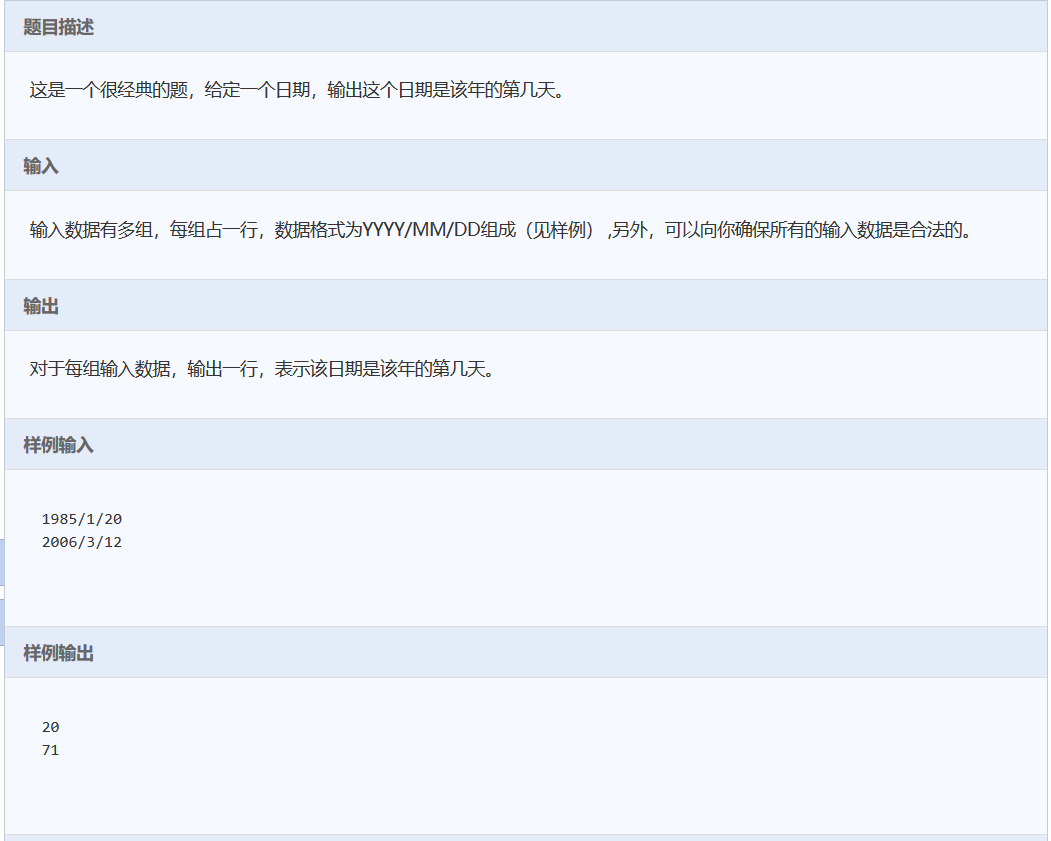#include<stdio.h>
int main()
{
int a={31,28,31,30,31,30,31,31,30,31,30,31};
int year,month,day;
while(scanf("%d/%d/%d",&year,&month,&day)!=EOF)
{
int sum=0;
for(int i=0;i<month-1;i++)
sum=sum+a[i];
sum=sum+day;
if((year%4==0&&year%100!=0)||year%400==0)
if(month>2)
sum++;
printf("%d\n",sum);
}
return 0;
}

//备注：其中在判断闰月加1天的时候必须当月份大于2时，才可以加1，否则将导致错误。

第2道：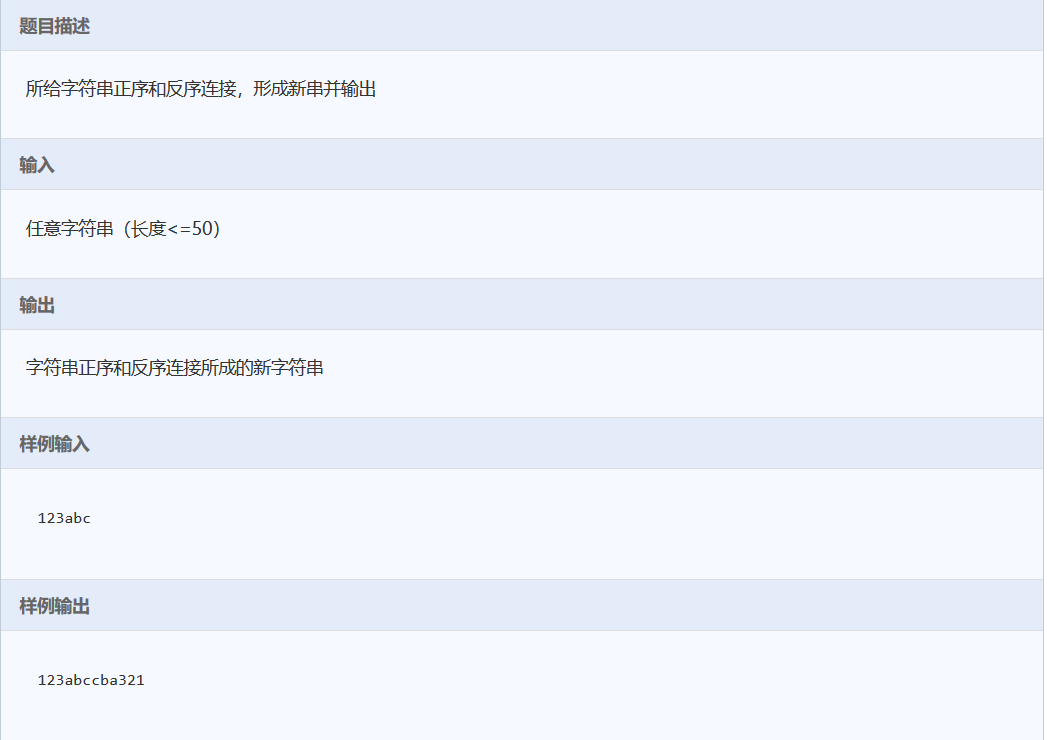#include<stdio.h>
#include<string.h>
int main()
{
int len;
int a,b;
char str;
char str2;
int i=0 ;
gets(str);
len=strlen(str);
a=len;
b=len;
strcpy(str2,str);
while(i<len)
{
str2[a++]=str[--b];
i++;
}
str2[a]='\0';
puts(str2);

return 0;
}

//备注：
//注意在编程过程中，当i为循环条件参数时，不可对其进行更改，解决办法是在定义一个变量对其备份。

第3道：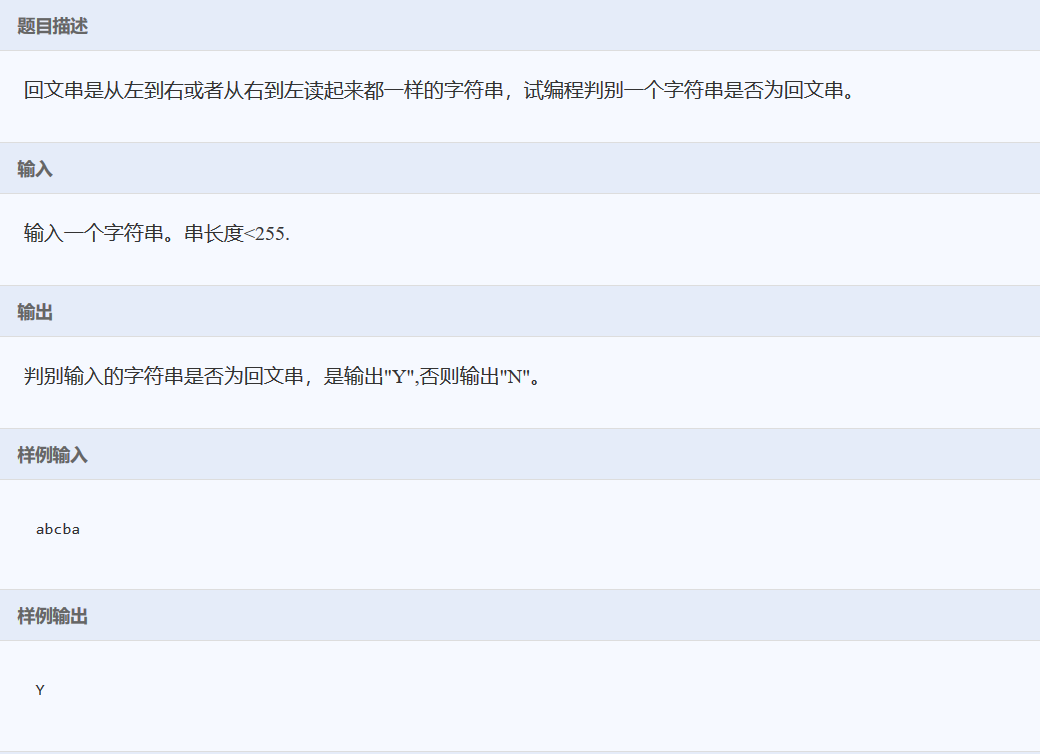#include<stdio.h>
#include<string.h>
int main()
{
int len;
char str;
int i=0 ;
int j=0;
gets(str);
len=strlen(str);
for(i=0,j=len-1;i<j;i++,j--)
{
if(str[i]!=str[j])
{
break;

}

}

if(i==j||i>j)
printf("Y");
else
printf("N");

return 0;
}

//备注：
//在写判断条件的时候一定要注意，要将字符串为单数和双数的情况都考虑进去，否则将导致错误。

第4道：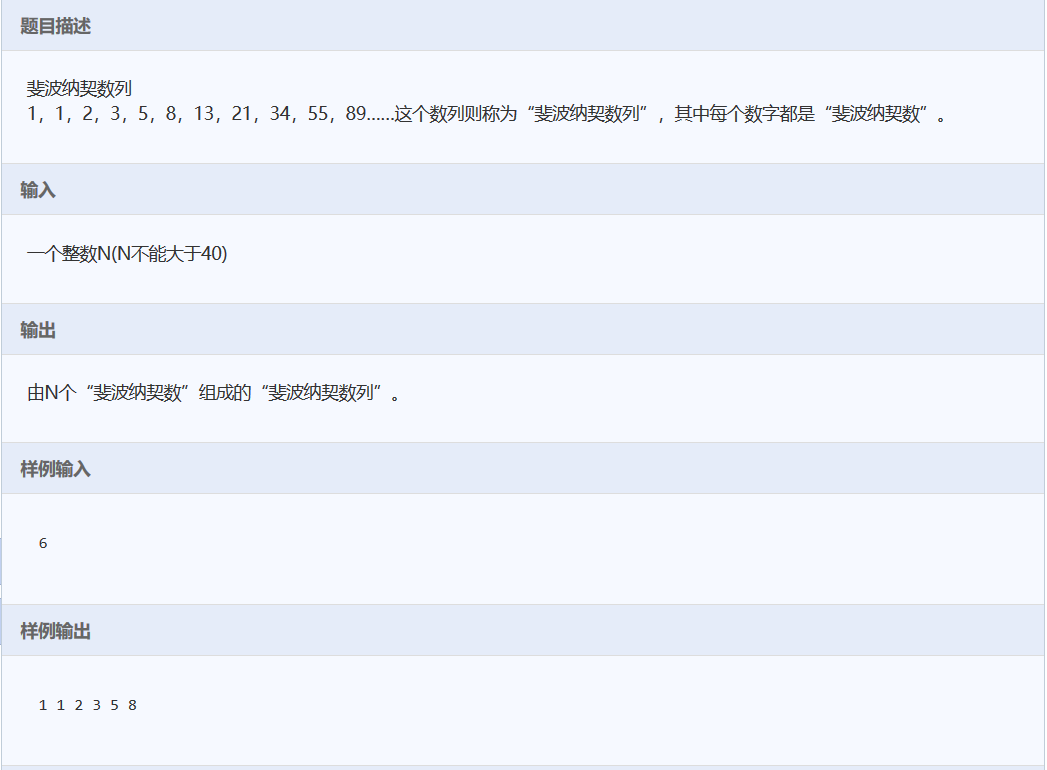#include<stdio.h>
int main()
{
int a;
int n;
int i;
scanf("%d",&n);
a=1;
a=1;
for (i=2;i<41;i++)
{
a[i]=a[i-2]+a[i-1];
}
for (i=0;i<n;i++)
{
printf("%d ",a[i]);
}

return 0;
}


第5道：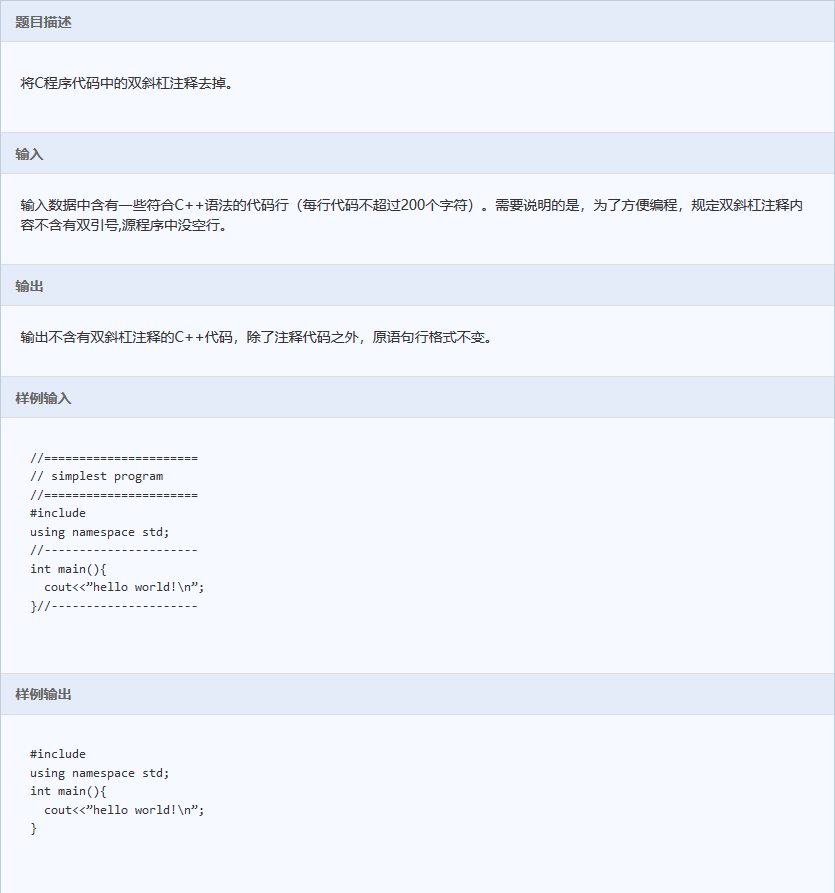#include<stdio.h>
#include<string.h>
int main()
{
int i;
int len;
char str;
while(gets(str)!=NULL)
{
len =strlen(str);
for (i=0;i<len-1 ;i++)
{
if(str[i]=='/'&&str[i+1]=='/')
str[i]='\0';
}
if(str!='\0')
printf("%s\n",str);
}
return 0;
}

//备注：
//这里涉及到多行输入的方法，以后遇到类似问题也可以这么干。

第6道：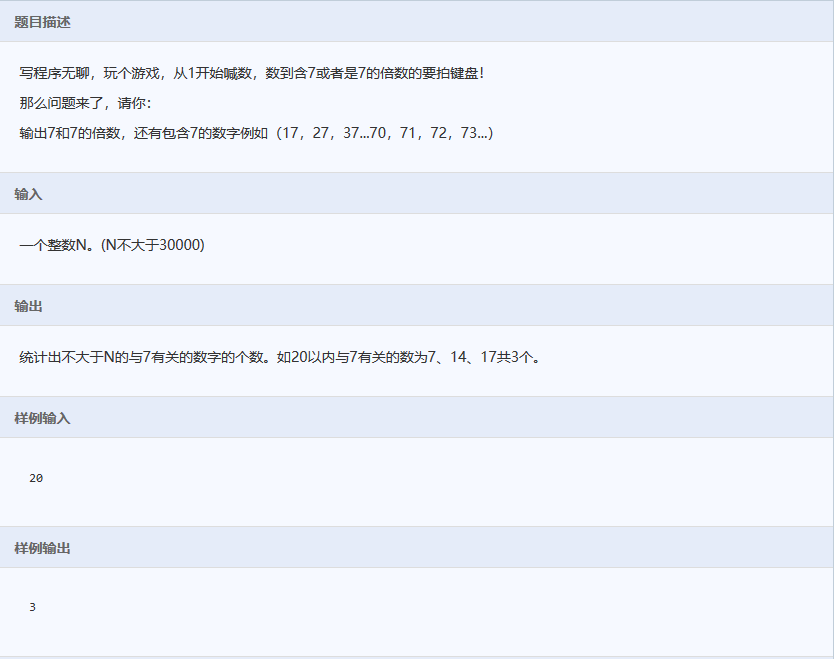#include<stdio.h>
#include<string.h>
int main()
{
int n;
int i;
int count=0;
int temp;
scanf("%d",&n);

for(i=1;i<=n;i++)
{
temp=i;
if(i%7==0)
{
count++;
}
else
{
while(temp)
{
if(temp%10==7)
{
count++;
break;
}
temp=temp/10;
}
}

}

printf("%d\n",count);
return 0;
}

//备注：
//这道题的难点不在于找出7的倍数，而在于找出不是7的倍数但包含7的数字，这里用了移位的思想，
//这个思想很重要，以后遇到需要对数据的每一位操作时候的题目也可以考虑用这种思想试试。


第7道：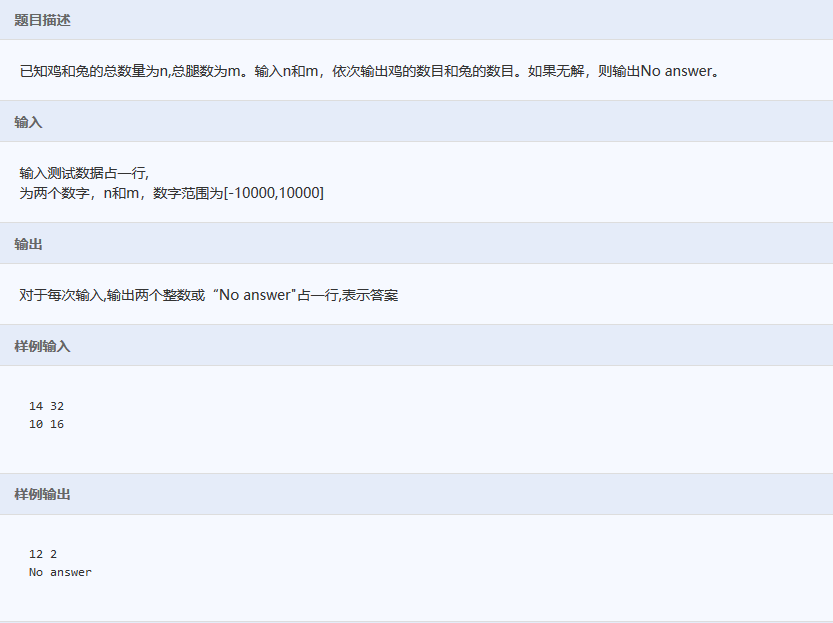#include<stdio.h>
int main()
{
int n,m;
int i=0;
int flag=0;
//a+b=n;
//2*a+4*b=m;
while( scanf("%d%d",&n,&m)==2)
{
flag=0;
for(i=0;i<=n;i++)
{
if((n-i)*4+2*i==m)
{
printf("%d %d\n",i,n-i);
flag=1;
}
}

if(flag==0)
{
printf("No answer\n");
}
}

return 0;
}

//备注：
//1.本题运用遍历思想。
//2.数据多组输入的方法需要掌握一下。

第8道：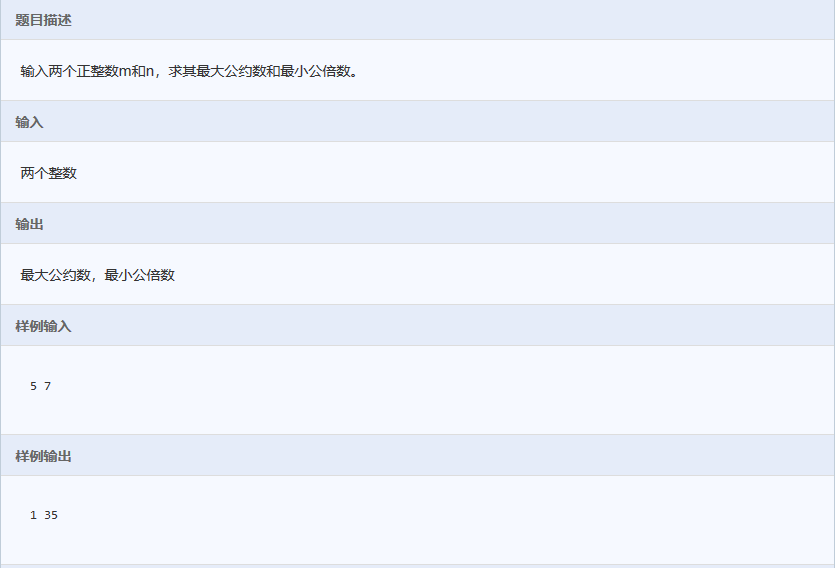#include<stdio.h>
int main()
{
int m,n;
int c;
int temp;
scanf("%d%d",&m,&n);
c=m*n;
if(m<n)
{
temp=m;
m=n;
n=temp;
}

while(m%n)
{
temp=m%n;
m=n;
n=temp;
}
printf("%d %d\n",n,c/n);
return 0;
}

//备注：
//”辗转相除法“求最大公约数，两数的和除以最大公约数求最小公倍数，这两种办法需要记住。


第9道：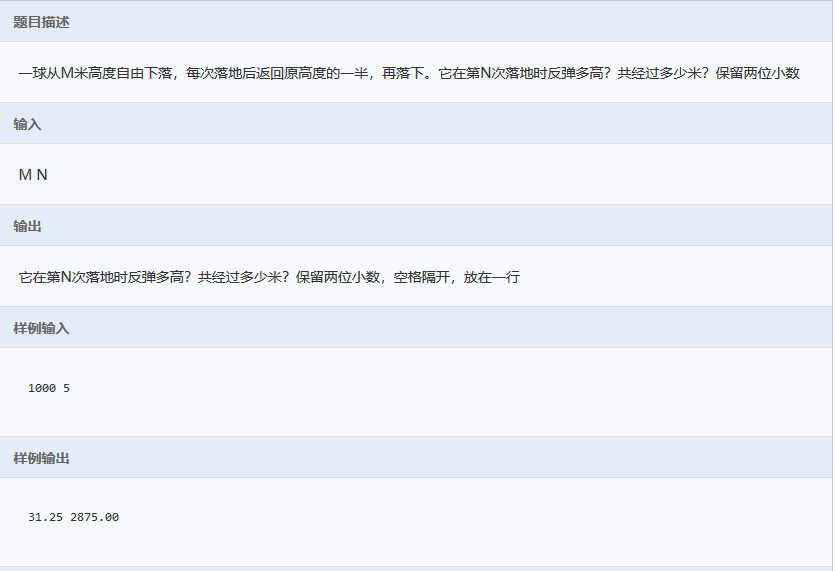#include<stdio.h>
int main()
{
int n;
double m,s;
int i;
scanf("%lf%d",&m,&n);
s=m;
for(i=0;i<n;i++)
{

m=m/2;
s=s+2*m;
}
s=s-2*m;
printf("%.2f %.2f\n",m,s);
return 0;
}

//备注：
//一定要读清楚题目要求，举例子画图，不然及其容易出错。

第10道：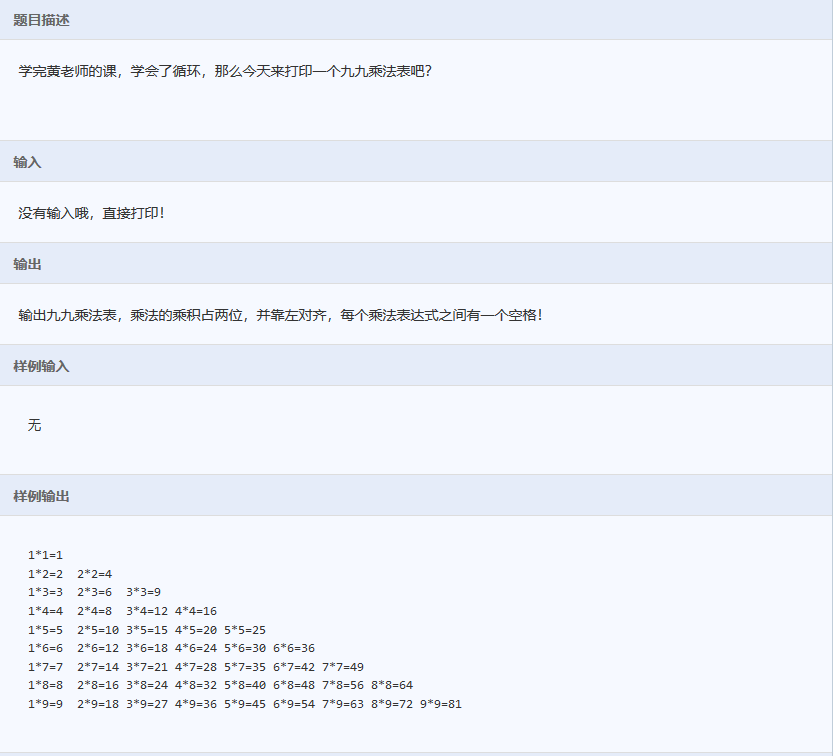#include<stdio.h>
int main()
{
int i,j;
for(i=1;i<=9;i++)
{
for (j=1;j<=i;j++)
{
printf("%d*%d=%-2d ",j,i,i*j);
}
printf("\n");
}
return 0;
}

//备注：
//该题看起来很简单，实际上很容易出错，错就错在打印格式，对齐方式等一定要注意。

第11道：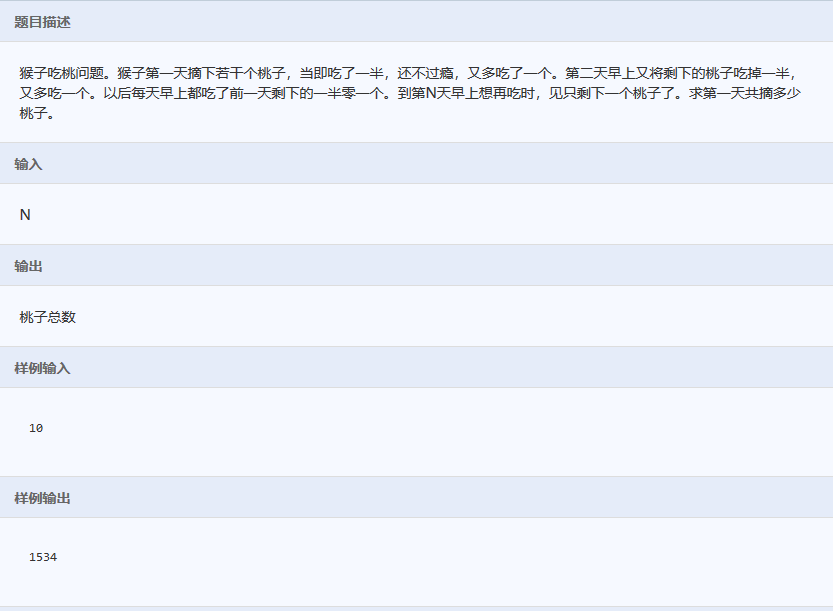#include<stdio.h>
int main()
{
int n;
int i;
int sum=1;
scanf("%d",&n);
for(i=1;i<n;i++)
{

sum=2*(sum+1);

}
printf("%d",sum);
return 0;
}
//备注：
//此题考察逆向思维，难点在于循环次数，为什么经历n天却只能循环n-次，
//原因是题目说了当天就吃了一半多一个，且最后一天还没吃就已经是1个了。所以只能循环n-1次。

第12道：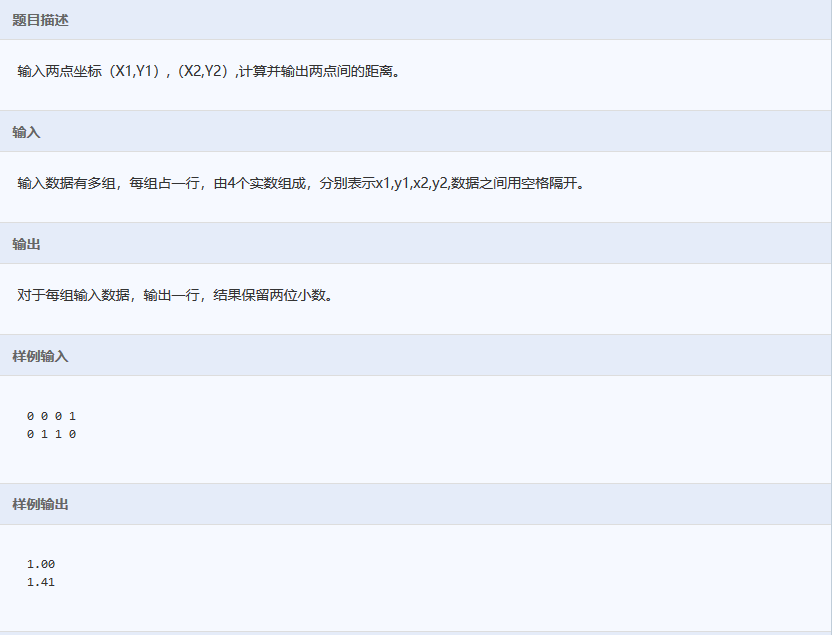#include<stdio.h>
#include<math.h>
int main()
{
int x1,y1,x2,y2;
double d=0;
while(scanf("%d%d%d%d",&x1,&y1,&x2,&y2)==4)
{
printf("%.2f\n",sqrt(pow(abs(x1-x2),2)+pow(abs(y1-y2),2)));
}
return 0;
}

//此题没有难度，只是为了熟悉常用的sqrt函数和pow函数。

第13道：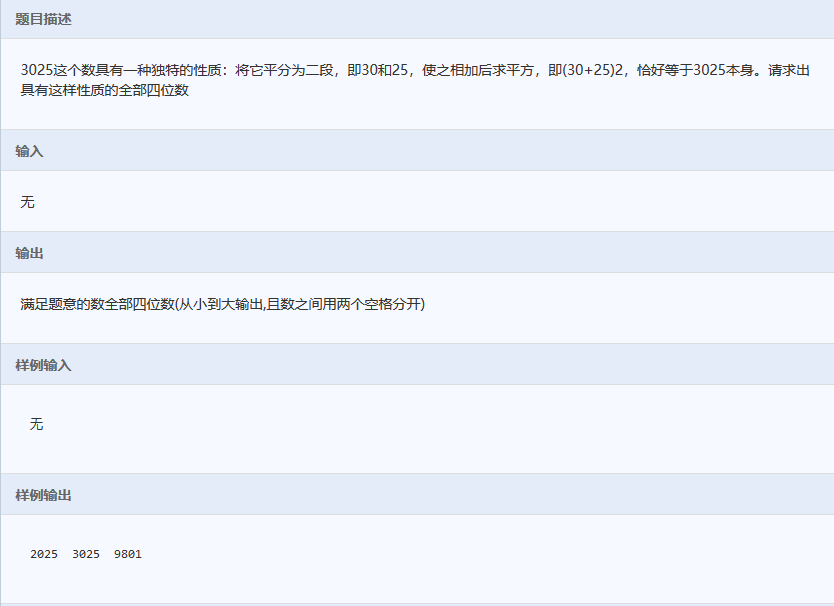#include<stdio.h>
#include<math.h>
int main()
{
int i=3025;
for(i=1000;i<9999;i++)
{

if((i/100+i%100)*(i/100+i%100)==i)
{
printf("%d  ",i);
}
}

return 0;
}

//备注：
//此题很简单，但是作者在做的时候有个犯了错误，原因是pow函数返回值是double类型，
//不可以和int类型的值进行比较，前玩不能用强转，转成int类型在进行比较
//原因是这样pow得到的结果会与真实结果有误差，因为会舍去小数部分。

第14道：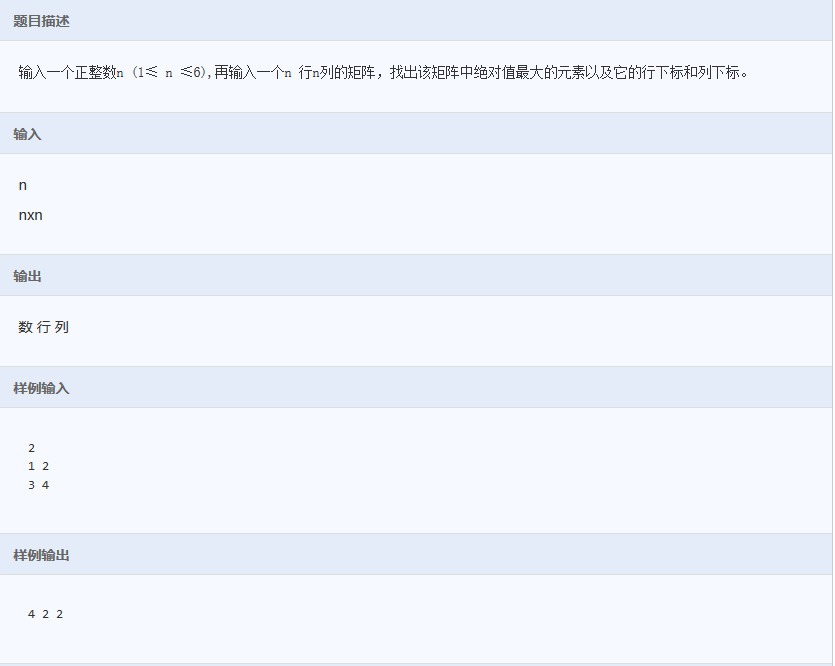#include<stdio.h>
#include<math.h>
int main()
{
int n;
int i,j;
int x,y;
int a;
int max=0;
scanf("%d",&n);
for(i=0;i<n;i++)
{
for(j=0;j<n;j++)
{
scanf("%d",&a[i][j]);
if(fabs(a[i][j])>fabs(max))
{
max=a[i][j];
x=i+1;
y=j+1;
}
}
}

printf("%d %d %d",max,x,y);
return 0;
}

//备注：
//主要注意多行输入以及下标需要加1。

第15道：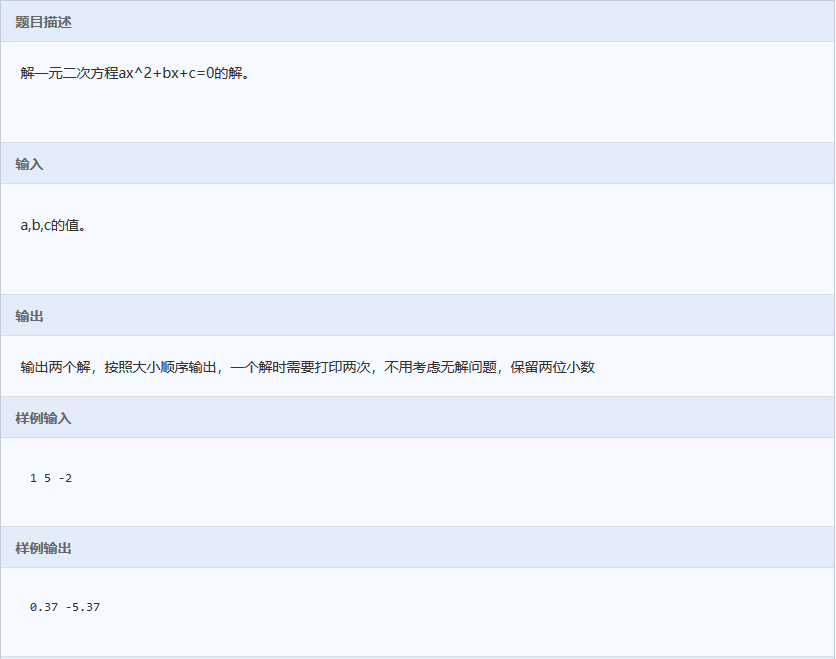#include<stdio.h>
#include<math.h>
int main()
{
double a,b,c;
double t;
double x1,x2;
scanf("%lf%lf%lf",&a,&b,&c);
t=pow(b,2)-4*a*c;
if(t>0)
{
x1=(-b+sqrt(t))/2*a;
x2=(-b-sqrt(t))/2*a;

}
else
{
x1=x2=-b/2*a;
}

printf("%.2f %.2f\n",x1,x2);
return 0;
}

//备注：
//此题需要注意的一点就是在同时接收int类型和char类型的时候，空格会影响接收
//解决办法就是在scanf的%d之间也加上同样多的空格。

第16道：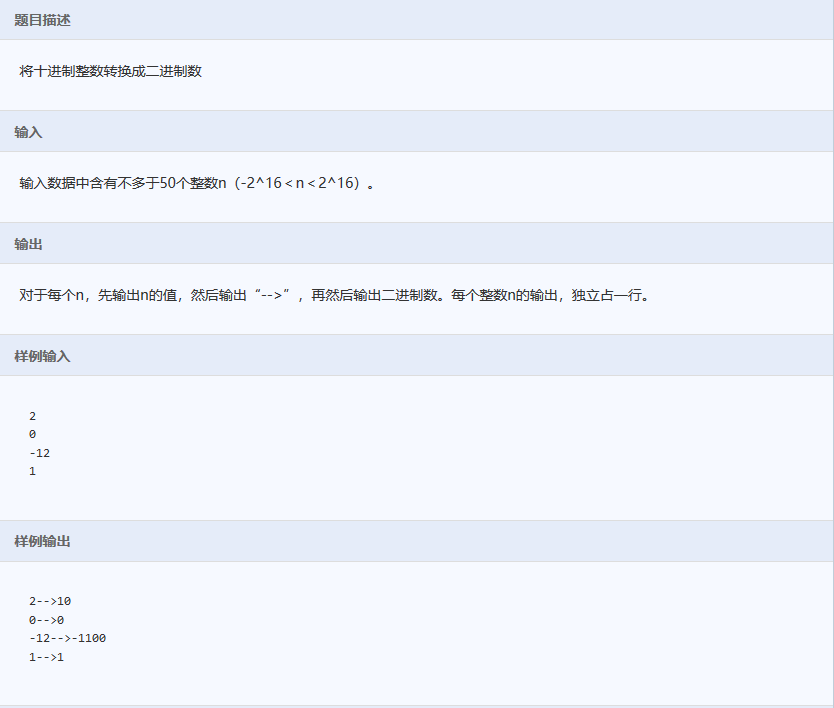#include<stdio.h>
int main()
{
int n;
int a;
int i=0;
while(scanf("%d",&n)==1)
{
i=0;
printf("%d-->",n);
if(n<0)
{
printf("-");
n=-n;
}
if(n==0)
{
printf("0");
}

while(n)
{
a[i++]=n%2;
n=n/2;
}

while(i--)
{
printf("%d",a[i]);
}
printf("\n");
}
return 0;
}

//备注：
//1.所有的进制转换都可以用这种方法
//2.这里最需要注意的是while（i--），这里while里面的i与while括号里面的i相差1.

第17道：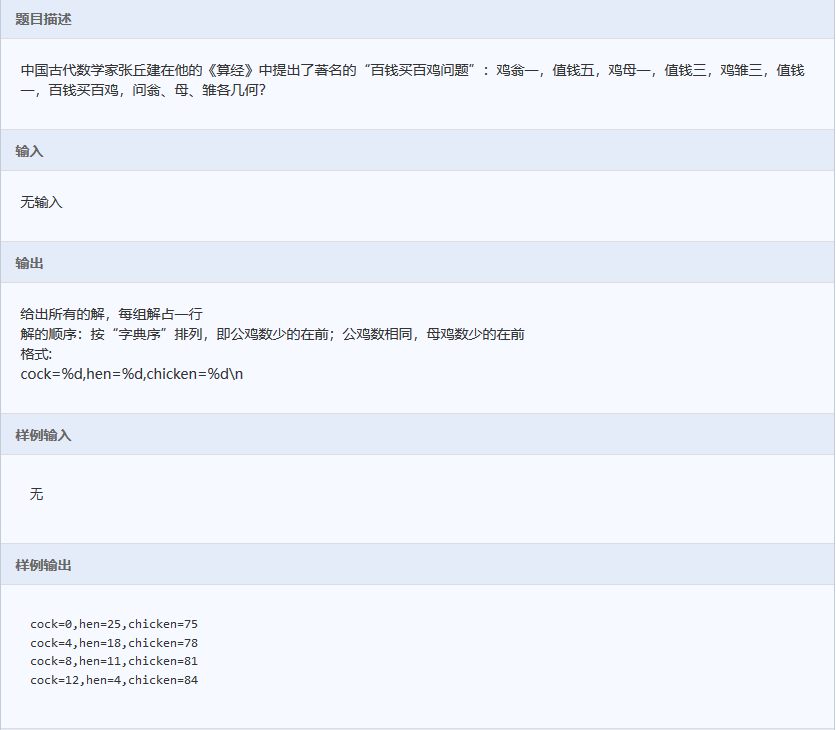#include<stdio.h>
int main()
{
int i,j,k;
for(i=0;i<=20;i++)
{
for(j=0;j<=34;j++)
{
for(k=0;k<=100;k++)
{
if(i+j+k==100&&5*i+3*j+k/3==100 &&k%3==0)
{
printf("cock=%d,hen=%d,chicken=%d\n",i,j,k);
}
}
}
}
return 0;
}

//备注：
//1.这里采用的是暴力算法，遍历思想；
//2.这里用嵌套的先后顺序，巧妙的解决了题目要求的字典排序输出。
//3.尽管用暴力算法，也要想办法优化代码，比如鸡翁不可能超过20只，
//鸡母不可能超过34只，大大减少循环次数。
//4.鸡雏的价钱不能简单的用k/3，因为例如k为10的话，结果也为3，所以要加上k%==0这个条件。

第18道：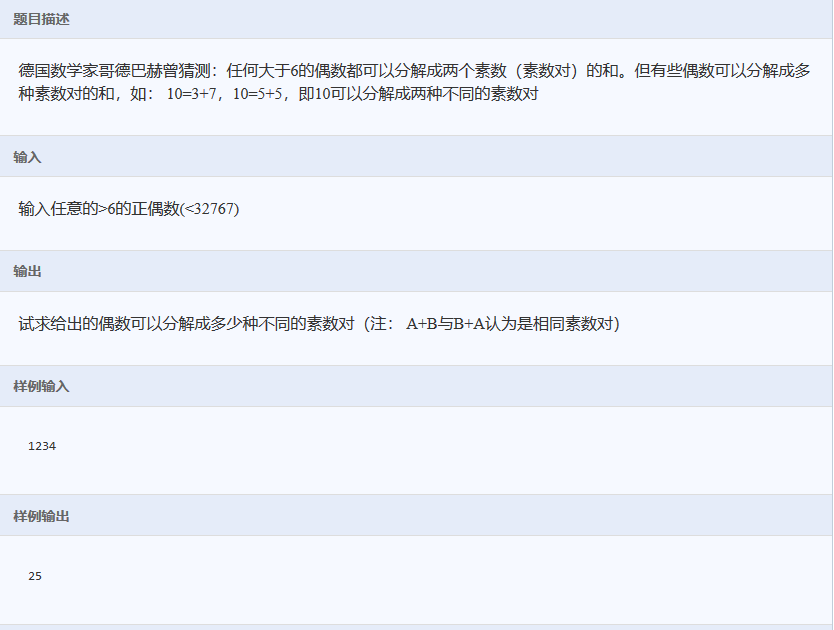#include<stdio.h>
#include<string.h>

int prime(int n)
{
int i;
int flag=0;
for (i=2;i<n;i++)
{
if(n%i==0)
{
flag=1;
break;
}
}
if(flag==1)
return 0;

return 1;
}
int main()
{
int n;
int i;
int count=0;
scanf("%d",&n);
for(i=2;i<=n/2;i++)
{
if(prime(i)&&prime(n-i))
{
count++;
}
}

printf("%d\n",count);
return 0;
}

//备注：
//1.一是要注意用函数对子功能进行封装，例如本题判断是不是素数。
//2.二是要注意重复情况的考虑，例如本体只遍历一般，将重复的情况去掉了。

第19道：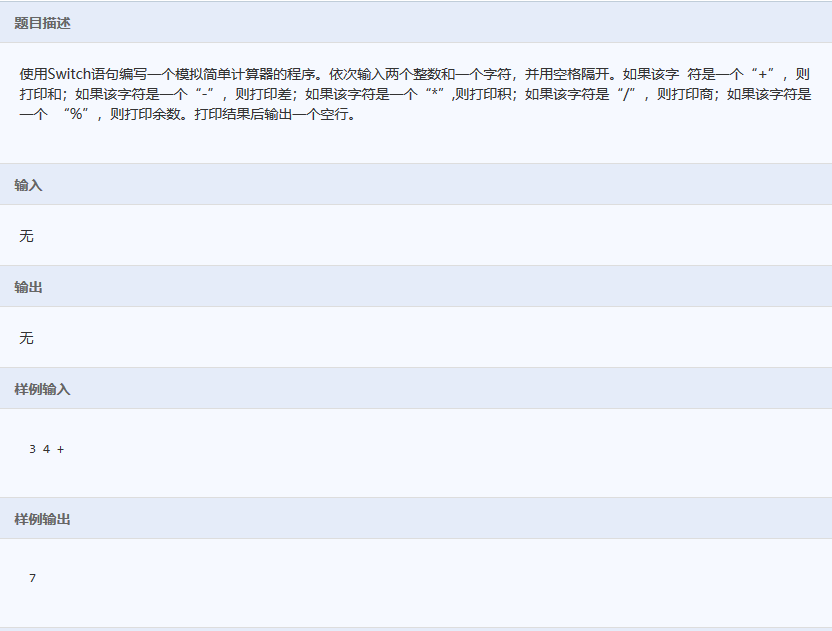#include<stdio.h>
#include<string.h>
int main()
{
int a,b;
char c;
scanf("%d %d %c",&a,&b,&c);
switch(c)
{
case '+':printf("%d",a+b);break;
case '-':printf("%d",a-b);break;
case '*':printf("%d",a*b);break;
case '/':printf("%d",a/b);break;
case '%':printf("%d",a%b);break;
default :break;
}
return 0;
}

//备注：
//这道题比较简单，主要是对switch的语法进行复习。

第20道：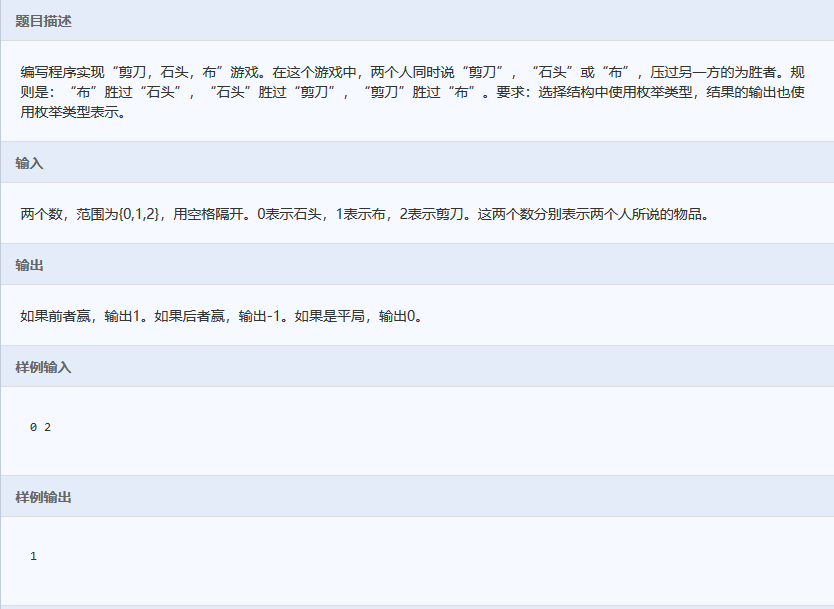#include<stdio.h>
enum hand{rock,paper,scissors};
enum ans{fail=-1,draw,win};
int main()
{
int a,b;
scanf("%d%d",&a,&b);
if(a==rock&&b==scissors||a==scissors&&b==paper||a==paper&&b==rock)
{
printf("%d\n",win);
}
else if(b==rock&&a==scissors||b==scissors&&a==paper||b==paper&&a==rock)
{
printf("%d\n",fail);
}
else
{
printf("%d\n",draw);
}
return 0;
}

//备注：本题主要是对枚举进行复习。


展开全文• C语言经典题目“小蜜蜂“代码小蜜蜂(选作)一只小蜜蜂在如下图所示的蜂窝上爬行。它爬行时，只能从一个格爬到相邻的大号格子中。例如，从 1 号格子可以爬到 2 号或者 3 号格子，从 2 号则可以爬到 3 号或者 4 号格子...
C语言经典题目“小蜜蜂“代码小蜜蜂(选作)一只小蜜蜂在如下图所示的蜂窝上爬行。它爬行时，只能从一个格爬到相邻的大号格子中。例如，从 1 号格子可以爬到 2 号或者 3 号格子，从 2 号则可以爬到 3 号或者 4 号格子。请问从一个格子 a 爬到一个格子 b 一共有多少种可行的路线。输入：分别是起始点 a 和终止点 b 的编号。( a 和 b 在 1~100 之间，且 a输出：程序：#include"stdio.h"#define N 50int main(){int b[N]={0};int c[N]={0};int i,j,k,n,a1,a2,t,l;b=c=1;scanf("%d%d",&a1,&a2);n=a2-a1+1;for(j=3;j<=n;j++){for(i=1;i{t=b[i]+c[i];if(l==1){c[i]=b[i]-1;l=0;}elsec[i]=b[i];b[i]=t;if(b[i]>=10){l=1;b[i+1]=b[i+1]+1;b[i]=b[i]%10;}}}k=N;while(b[--k]==0){}for(i=k;i>=1;i--)printf("%d",b[i]);printf("\n");return 0;}#include int main(){long double num1,num2,num3=0;int a=0,b=0,i;scanf("%d %d",&a,&b);num1=1;num2=2;if(b-a==1)printf("1\n");else if(b-a==2)printf("2\n");else{for(i=3;i<=b-a;i++){num3=num1+num2;num1=num2;num2=num3;}printf("%.0lf\n",num3);}return 0;}
展开全文• 很好很经典题目，一些基础好一点的，想提高下的可以下来看看，初学者也可以看看，学以致用
• 文档包含C语言，简答(包含一些面试高概率问题)、程序分析题(包含编程中常见错误现象，内存越界，非法操作，段错误分析等)、编程题(30道编程题，涵盖了面试常见题型，以及C语言经典程序)。学习，检测，准备面试，都是...
• ## C语言循环结构经典编程题

千次阅读 多人点赞 2020-06-20 11:38:05
1.求和S。 题目描述题目内容：求s=1+(1+2)+…+(1+2+3+…+n)的值，其中n由键盘...3 找出最小值 (20分)本要求编写程序，找出给定一系列整数中的最小值。 4 奇偶分家 (10分)给定N个正整数，请统计奇数和偶数各有多少个？
1  求和S。
题目描述题目内容：求s=1+(1+2)+…+(1+2+3+…+n)的值，其中n由键盘输入。
输入样例：3
输出样例：10
输入样例：8
输出样例：120
输入样例：0
输出样例：Error！
输入样例：-1
输出样例：Error！
题目运行时间限制： 500ms题目运行内存限制： 32000kb
代码：
#include<stdio.h>
main(){
int s=0,i,n,a=0,b=0;
scanf("%d",&n);
if(n<1) printf("Error!");
else
{
for (i=0;i<n;i++)
{
++a;
b+=a;
s+=b;
}
printf("%d",s);
}
}
2  追查车号。题目描述题目内容：一辆卡车违反交通规则，撞人逃逸。现场三人目击事件，但都没有记住车号，只记下车的一些特征。甲说：牌照的前两位数字是相同的；乙说：牌照的后两位数字是相同的；丙是位数学家，他说：四位的车号正好是一个整数的平方。请根据以上线索求出车号。输出格式：The number is ****。测试用例用例1分数： 20错误提示：题目运行时间限制： 500ms题目运行内存限制： 32000kb.
代码：
#include<stdio.h>
main()
{
int a,b,i,j;
for (i=1;i<10;i++)
for(j=0;j<10;j++)
{
a=i*1000+i*100+j*10+j;
for(b=10;b<100;b++)
if(b*b==a)
printf("The number is %d",a);
}
}
3 找出最小值 (20分)本题要求编写程序，找出给定一系列整数中的最小值。
输入格式：输入在一行中首先给出一个正整数n，之后是n个整数，其间以空格分隔。
输出格式：在一行中按照“min = 最小值”的格式输出n个整数中的最小值。
输入样例：4 -2 -123 100 0
输出样例：min = -123
代码：
#include<stdio.h>
int main()
{
int n,i,a,b;
scanf ("%d %d",&n,&a);
for(i=1;i<n;i++){
scanf (" %d",&b);
if(a>b) a=b;
}
printf ("min = %d",a);
}
4 奇偶分家 (10分)给定N个正整数，请统计奇数和偶数各有多少个？
输入格式：
输入第一行给出一个正整N（≤1000）；第2行给出N个非负整数，以空格分隔。
输出格式：
在一行中先后输出奇数的个数、偶数的个数。中间以1个空格分隔。
输入样例：
9
88 74 101 26 15 0 34 22 77
输出样例：
3 6
代码：
#include<stdio.h>
int main()
{
int N,i,j=0,o=0,a;
scanf("%d\n",&N);
for (i=0;i<N;++i){
scanf("%d",&a);
if(a%2) j+=1;
else o+=1;
}
printf("%d %d",j,o);
}


展开全文• 猴子吃桃问题:猴子第一天摘下若干个桃子,马上吃了一半,没有吃饱,...这样的我们通常采用倒推的方法,就是已知第十天的桃子数量,反过来求第一天的. 我们知道第十天为1 所以第九天就为 (1 + 1) * 2 = 4 第八天就为 (4...
• 这些事C语言编程中比较经典的试题，希望大家仔细看看，对以后的编程有较大的帮助。
• （2）如果学习过C语言，在学习其他高级编程语言时更加轻松。比如c++C#等。 （3)C的程序来运行效率更高，更快，程序可以很简洁。 （4）c语言网上资料很多，学习起来较为容易。 （5）找编程开发方面的工作时，C语言...
• 1.题目：将一个正整数分解质因数。例如：输入90,打印出90=2*3*3*5。 /*分解质数*/ #include<iostream> using namespace std; void Divide(int num) { int numcopy =num; for(int i=2;i<=numcopy...
• 这个是C语言编程的一百道经典题目。有详细的解答，很利于提高C的水平值得一看
• 题目：有1、2、3、4个数字，能组成多少个互不相同且无重复数字的三位数？都是多少？ ============================================================== 【程序2】 题目：企业发放的奖金根据利润提成。利润(I)低于...
• 经典C语言程序设计习题，共100，内附有答案与习题简略解析； 题目：有1、2、3、4 个数字，能组成多少个互不相同且无重复数字的三位数？都是多少？ 1.程序分析：可填在百位、十位、个位的数字都是1、2、3、4。...
• 这是大学C程序必做的题C语言编程经典100例【程序1】题目：有1、2、3、4个数字，能组成多少个互不相同且无重复数字的三位数？都是多少？程序分析：可填在百位、十位、个位的数字都是1、2、3、4。组1.1.程序分析：可填...
• ## 100道经典的c语言面试题

万次阅读 多人点赞 2018-07-26 19:19:45
100 条经典C语言笔试题目   题目来源： 1、中兴、华为、慧通、英华达、微软亚洲技术中心等中 外企业面试题目； 2、C 语言面试宝典(林锐《高质量编程第三版》)。 说明： 1、部分C 语言面试中可能会参杂部分和...
• 在此整理出之前做的一些题目，所有函数包含在同一个头文件中，可在main函数中自行测试，都是些经典题目，供新手老手参阅。转载标明出处，谢谢。// mywork.c // data_struct // // Created by sdd on 17/8/22. // ...
• c语言编程的一些基础题目，帮助初学者学习C语言。
• C语言上机100题，包含改错题，填空题，编程题题目非常经典，非常实用。
• 实用标准文案 实用标准文案 文档 文档 关键字C 经典C源程序100例 程序1 题目古典问题有一对兔子从出生后第 3个月起每个月都生一对兔子小兔子长到第 三个月后每个月又生一对兔子假如兔子都不死问每个月的兔子总数为...
• 后来回到学校，在网上搜索，原来这些都是嵌入式工程师的经典面试题目，很多网站上都可以找得到。现把他贴出来，附上网上的答案，跟大家分享，因为这些实在太经典了。 预处理器（Preprocessor） 1 . 用预处理指令...
• 试卷名称： Java 经典面试带答案试卷描述： java 笔试题目、招聘笔试、微信考试、在线考试试卷链接：试卷限时： 50 分一.单项选择分值：分是否题目乱序：是是否选项乱序：是是否可回溯：是难度：中[单选 ]...
• 1. 顺序存储的n个整数，编程输出最大的前k个 #include<stdio.h> #define N 10 void sort(int *a,int n) { int i,j,t; for(i=0;i<n-1;i++) for(j=0;j<n-i-1;j++) if(a[j]<a[j+1]) { t=a......

# c语言编程题经典题目c语言 订阅